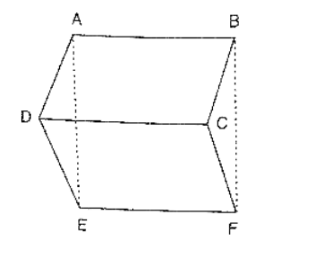# In figure, ABCD, ABFE and CDEF are parallelograms. Prove that ar(ΔADE) = ar(ΔBCF).Question:

In figure, ABCD, ABFE and CDEF are parallelograms. Prove that ar(ΔADE) = ar(ΔBCF).Solution:

Given that

ABCD is parallelogram ⇒ AD = BC

CDEF is parallelogram ⇒ DE = CF

ABFE is parallelogram ⇒ AE = BF

Thus, in Δs ADF and BCF, we have

AD = BC, DE = CF and AE = BF

So, by SSS criterion of congruence, we have